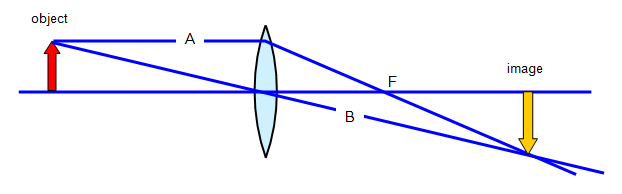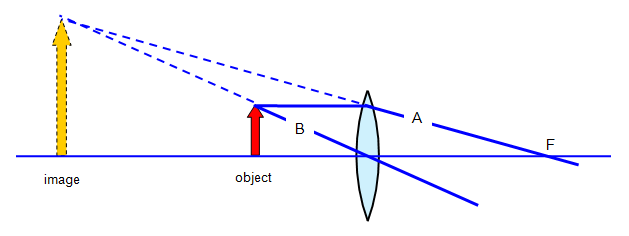# Finding the image made by a lens by a scale diagram

The image can be found by a diagram as shown below.
The object and focal length are drawn to scale and then three rays are drawn from the object:
(a) the axis;
(b) a ray from the top of the object parallel to the axis (A);
(c) a ray from the top of the object passing through the centre of the lens (B).

Where rays (b) and (c) cross is the top of the image.

Two examples of this are shown below.

Convex lens with the object further from the lens than its focal lengthAfter passing through the lens ray A will go through the principal focus (F).

Convex lens with the object closer to the lens than its focal lengthWORD VERSION AVAILABLE ON THE SCHOOLPHYSICS USB